Next: FEW-NUCLEON SYSTEMS Up: Dynamical (Chiral) Symmetry Breaking Previous: Pion-Pion Lagrangian

### Nucleon-Pion Lagrangian

We are now ready to consider another set of composite particles, the nucleons. We know that there are two nucleons in Nature, of almost equal mass, the neutron and the proton, so they can be combined into the iso-spinor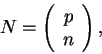(40)

where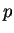and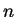are the Dirac four-spinors of spin 1/2 particles. We have already attributed the isospin projections to quarks, Eq. (26), by placing within the quark iso-spinor the quark up up and the quark down down (sounds logical?). Since the proton is made of the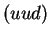quarks, and the neutron of the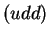quarks, their isospin projections are therefore determined as in Eq. (40). In nuclear structure physics one usually uses the opposite convention, attributing the isospin projection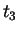=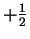to a neutron, in order to make most nuclei to have positive total isospin projections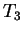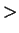0. All this is a matter of convention; one could as well put the quark up down and the quark down up - the physics does not depend on that.

Anyhow, the nucleons contain not only the three (valence) quarks, but also plenty of gluons, and plenty of virtual quark pairs, and we are unable to find what exactly this state is. Therefore, here we follow the general strategy of attributing elementary fields to composite particles. Before we arrive at sufficiently high energies, or small distances, at which the internal structure of composite objects becomes apparent, we can safely live without knowing exactly how the composite objects are constructed.

As usual, having defined elementary fields of particles that we want to describe, we also have to postulate the corresponding Lagrangian density. And as usual, we do that by writing a local function of fields that is invariant with respect to all conserved symmetries. When we have the nucleon and pion fields at our disposal, and we want to construct the Lorentz and chiral invariant Lagrangian density, the answer is: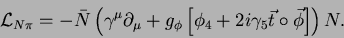(41)

If you are not tired of this game of guessing the right Lagrangian densities, you may wonder why the meson fields (within the square brackets) appear in this particular form. To really see this, we have to recall more detailed properties of the chiral group SU(2)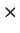SU(2). Its generators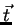and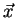in the spinor representation are given by Eq. (28), however, when more than one quark is present, we have to use the analogous generators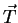and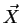that are sums of's and's for all quarks. In particular, the meson fields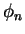belong to the vector representation of SU(2)SU(2). Then, according to identification (35) and (37), the first three components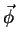form the isovector pion field, and the fourth component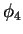is an isoscalar. This fixes the transformation properties ofwith respect to the iso-rotations, given by infinitesimal transformation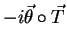. Since these rotations have identical form as the real rotations in our three-dimensional space, we do not show them explicitly. On the other hand, the transformation properties ofwith respect to the chiral rotations, given by infinitesimal transformation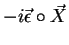, are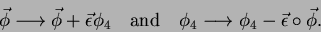(42)

There is no magic in this expression - one only has to properly identify generators of the O(4) group with generatorsand. This is unique, once we fix which components (1,2,3 in our case) transform under the action of. Under the chiral rotation about the same angle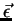, the nucleon fields transform by infinitesimal transformation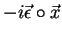, within the spinor representation of Eq. (28), i.e.,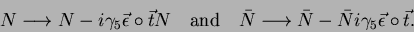(43)

It is now a matter of a simple algebra to verify that Lagrangian density (41) remains invariant under chiral rotations of fields (42) and (43). Note that the first term in Eq. (41) is separately chiral invariant, so we could multiply the second term by an arbitrary constant.

We can now proceed with the transformation to better variables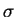and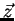, given by Eq. (35), which gives,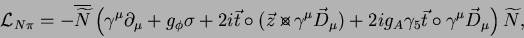(44)

where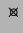denotes vector product in the iso-space. Covariant derivatives of pion fields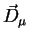are defined as in Eq. (39), and the chiral-rotated nucleon field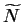is defined as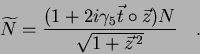(45)

There are several fantastic results obtained here. First of all, the nucleon mass term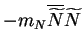appears out of nowhere, and the nucleon mass,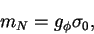(46)

is given by the chiral-symmetry-breaking value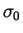of thefield. In principle, we could begin by including the nucleon mass term already in the initial Lagrangian density (41). This is not necessary - the nucleon mass results from the same chiral-symmetry-breaking mechanism that pushes scalar mesons up to high energies. Second, the third term in Eq. (44) gives the coupling of nucleons to mesons, and in the potential approximation it yields the long-distance, low-energy tail of the nucleon-nucleon interaction, i.e., the one-pion-exchange (OPE) Yukawa potential Yuk35. Derivation of this potential from Lagrangian density (44) requires some fluency in the methods of quantum field theory, so we do not reproduce it here. Suffice to say, that the OPE potential appears as naturally from exchanging pions, as the Coulomb potential appears from exchanging photons via the electron-photon coupling term in Eq. (16). Last but not least, the last term in Eq. (44) gives the axial-vector current that defines the weak coupling of nucleons to electrons and neutrinos. From where phenomena like the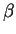decay can be derived. [This term is an independent chiral invariant, so again we could put a separate coupling constant there; experiment gives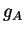=1.257(3).]Next: FEW-NUCLEON SYSTEMS Up: Dynamical (Chiral) Symmetry Breaking Previous: Pion-Pion Lagrangian
Jacek Dobaczewski 2003-01-27